Function Repository Resource:

# DominatingIntegerPartitionQ

Determine if one integer partition dominates another

Contributed by: Wolfram Staff (original content by Sriram V. Pemmaraju and Steven S. Skiena)
 ResourceFunction["DominatingIntegerPartitionQ"][p,q] yields True if integer partition p dominates integer partition q and False otherwise.

## Details

For two integer partitions p={p1,,pk} and q={q1,,kl} of some positive integer n, p is said to dominate q if the sum of the t largest parts of p is greater than or equal to the sum of the t largest parts of q, p1++ptq1++qt, for all t1, where the partition with fewer parts is padded with zeros at the end.
Partitions p and q can be incomparable with respect to dominance.

## Examples

### Basic Examples (2)

Since 5+1<4+3, the first partition does not dominate the second:

 In:=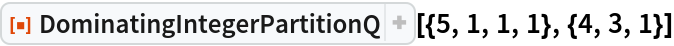Out=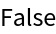The first partition dominates the second, since 44, 4+44+3 and 4+44+3+1:

 In:=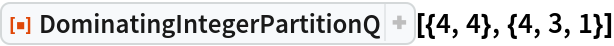Out=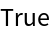### Applications (1)

Display the domination lattice on integer partitions, using the resource function HasseDiagram:

 In:=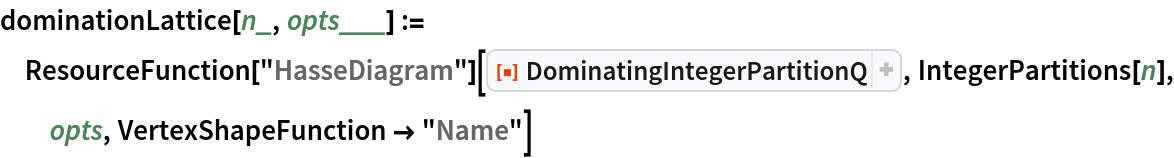In:=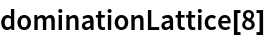Out=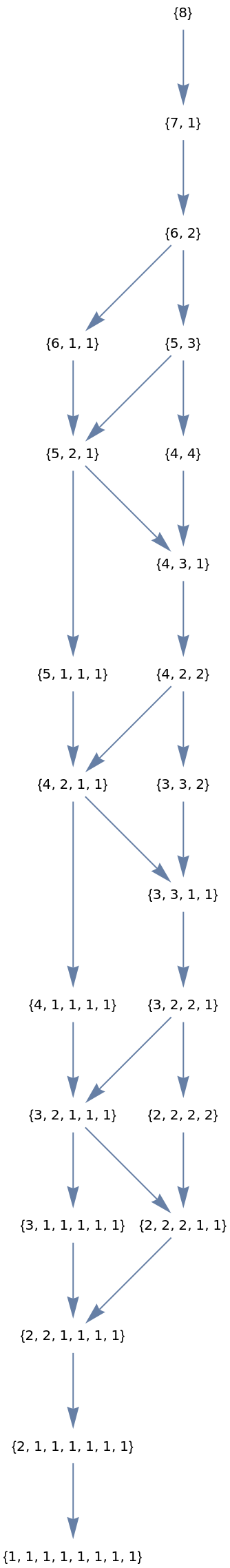### Properties and Relations (3)

DominatingIntegerPartitionQ[p,q] being False does not imply that DominatingIntegerPartitionQ[q,p] is True:

 In:=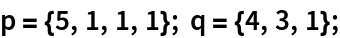In:=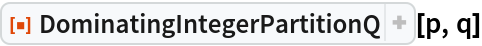Out=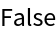In:=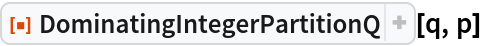Out=In this case, this is because the first element of q is smaller than the first element of p:

 In:=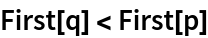Out=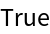DominatingIntegerPartitionQ[p,q] and DominatingIntegerPartitionQ[q,p] can both yield True for certain p and q:

 In:=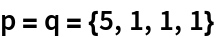Out=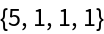In:=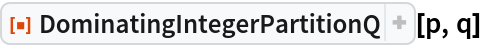Out=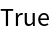In:=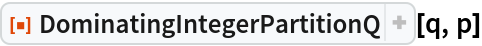Out=Among the partitions of n, {n} is always the largest and {1,,1} is the smallest:

 In:=Out=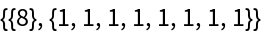## Version History

• 1.0.1 – 31 January 2022
• 1.0.0 – 28 July 2020